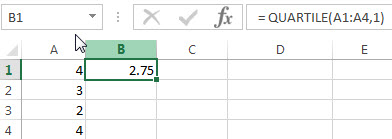# Excel QUARTILE Function

This post will guide you how to use the QUARTILE function with syntax and examples in Microsoft excel.

### Description

The Excel QUARTILE function returns the quartile from a supplied range of values. This function has been replaced with QUARTILE.EXC function and QUARTILE.INC function.

The QUARTILE function is a build-in function in Microsoft Excel and it is categorized as a Statistical Function.

The QUARTILE function is available in Excel 2016, Excel 2013, Excel 2010, Excel 2007, Excel 2003, Excel XP, Excel 2000, Excel 2011 for Mac.

### Syntax

The syntax of the QUARTILE function is as below:

`= QUARTILE (array,quart)`

Where the QUARTILE function arguments are:

• Array – This is a required argument.  The array or range of cells that contain numeric values for which you want to get the quartile value.
• Quart – This is a required argument. The quartile value that you want to return.  The value can be set in the following 5 values.
 If quart equals QUARTILE returns 0 Minimum value 1 First quartile (25th percentile) 2 Median value (50th percentile) 3 Third quartile (75th percentile) 4 Maximum value

Note:

• If the quart value is not an integer, and the QUARTILE function will truncate it.
• If the quart argument is empty, the QUARTILE function will return the #NUM! Error.
• If the quart value is small than 0 or greater than 4, the QUARTILE function will return the #NUM! Error.

### Excel QUARTILE Function Examples

The below examples will show you how to use Excel QUARTILE Function to get the quartile from a range of cells.

Example 1:  to get the first quartile of a given range of cells (A1:A4), using the following formula:

`= QUARTILE(A1:A4,1)`Related Posts

Excel DVAR Function

This post will guide you how to use Excel DVAR function with syntax and examples in Microsoft excel. Description The Excel DVAR Function will get the variance of a population based on a sample of numbers in a column in ...

Excel DSUM Function

This post will guide you how to use Excel DSUM function with syntax and examples in Microsoft excel. Description The Excel DSUM Function will add the numbers in a column or database that meets a given criteria. And so you ...

Excel DSTDEVP Function

This post will guide you how to use Excel DSTDEVP function with syntax and examples in Microsoft excel. Description The Excel DSTDEVP returns the standard deviation of a population based on the entire population of numbers in a column or ...

Excel DSTDEV Function

This post will guide you how to use Excel DSTDEV function with syntax and examples in Microsoft excel. Description The Excel DSTDEV returns the standard deviation of a population based on a sample of numbers in a column or database ...

Excel DMIN Function

This post will guide you how to use Excel DMIN function with syntax and examples in Microsoft excel. Description The Excel DMIN returns the minimum value from a database or a column of a list that matches the specified conditions. ...

Sidebar